# 数学建模之差分方程模型详解

原创不易，转载请联系作者并注明出处# 一阶线性常系数差分方程的平衡点及其稳定性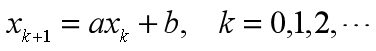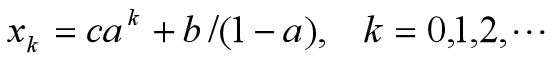c= x0-b/(1-a)由初始值x0 和a、b确定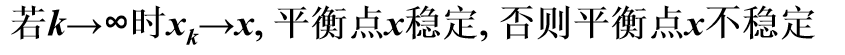平衡点稳定的充要条件是 |a|<1

# 高阶线性常系数差分方程的平衡点及其稳定性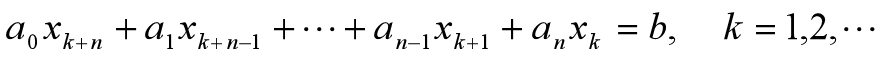特征方程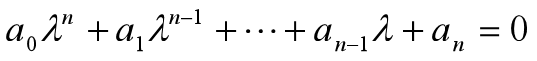特征根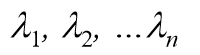平衡点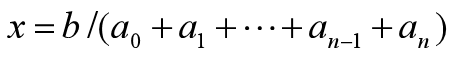差分方程的解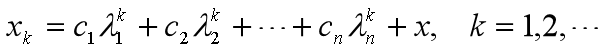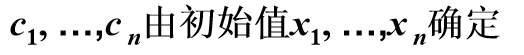平衡点稳定的条件: 所有特征根的模小于1

# 一阶线性常系数差分方程

## 濒危物种(Florida 沙丘鹤)的自然演变和人工孵化

### 问题提出

Florida沙丘鹤属于濒危物种，它在较好自然环境下，年均增长率仅为1.94%，而在中等和较差环境下年均增长率分别为 -3.24% 和 -3.82%，如果在某自然保护区内开始有100只鹤，建立描述其数量变化规律的模，并作数值计算。

### 模型建立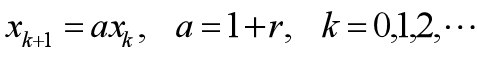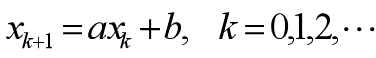那么可以得到

### 模型求解

>>X0=100;
>> r=0.0194;
>> n=20;
>>Xn=(1+r)^n*X0

 function x=sqh(n,r)
x(1)=100;
for k=1:n
x(k+1)=(1+r)*x(k);
end

>>xn＝sqh(20,0.0194)
xn =

Columns 1 through 6

100.0000  101.9400  103.9176  105.9336  107.9888  110.0837

Columns 7 through 12

112.2194  114.3964  116.6157  118.8780  121.1843  123.5353

Columns 13 through 18

125.9318  128.3749  130.8654  133.4042  135.9922  138.6305

Columns 19 through 21

141.3199  144.0615  146.8563


>>k=0:20；
>>y1= sqh(20,0.0194);
>>plot(k,y1)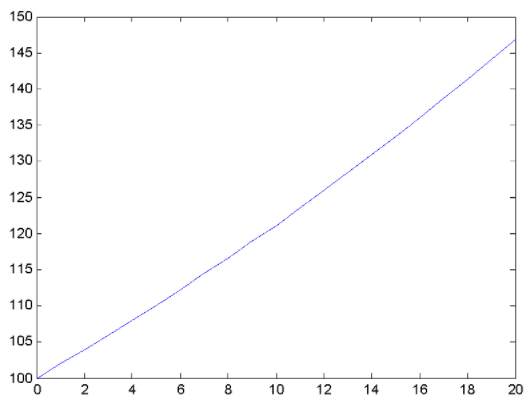在同一坐标系下画图

>> k=0:20;  %一个行向量
>> y1= sqh(20, 0.0194);
>> y2= sqh(20, -0.0324);
>> y3= sqh(20, -0.0382);
>> plot(k,y1,k,y2,':',k,y3,'r')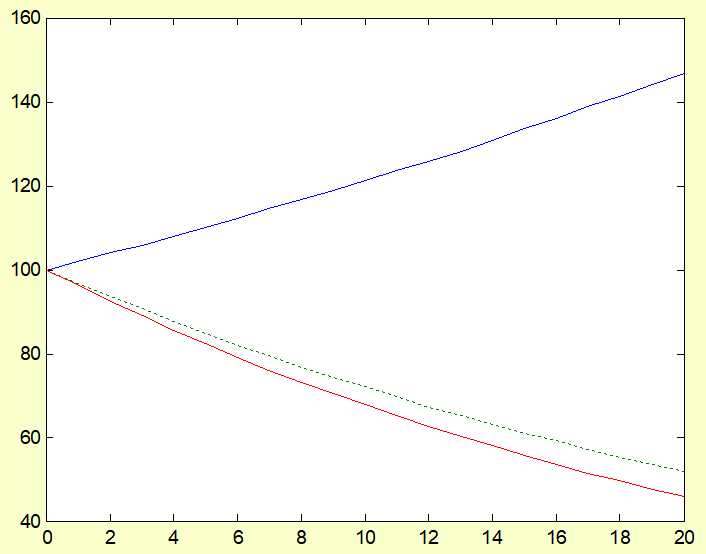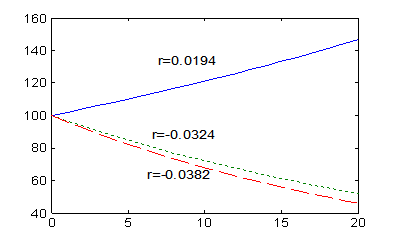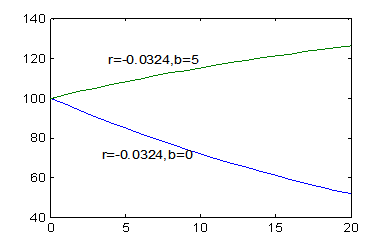### 结果分析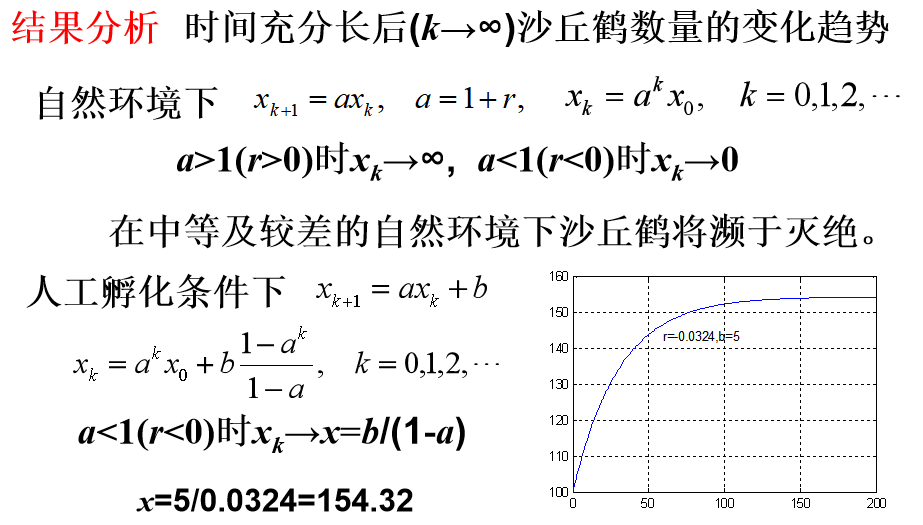# 高阶线性常系数差分方程

## 一年生植物的繁殖

### 模型建立

设一棵植物平均产种数为c，种子能够活过冬天的比例为b，活过冬天的那些种子在来年春季发芽的比例为，未能发芽的那些种子又活过一个冬天的比例仍为b， 在下一年春季发芽的比例为

~第k年的植物数量，设今年种下(并成活)的数量为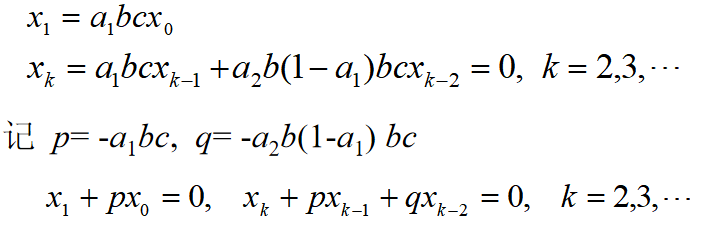寻找形如的解

### 模型求解

matlab建立如下函数：

function x=zwfz(x0,n,b)
c=10;a1=0.5;a2=0.25;
p=a1*b*c;q=a2*b*(1-a1)*b*c;
x(1)=x0;
x(2)=p*x(1);
for  k=3:n
x(k)=p*x(k-1)+q*x(k-2);
end

>> k=0:20;
>> y1=zwfz(100,21,0.18);
>> y2=zwfz(100,21,0.19);
>> y3=zwfz(100,21,0.20);
>> plot(k,y1,k,y2,':',k,y3,'r')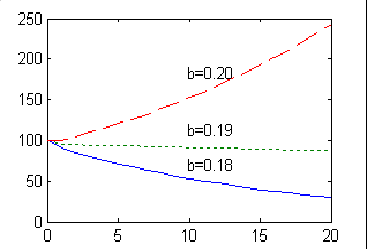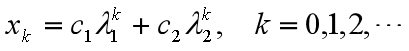常数c1, c2由x0, x1确定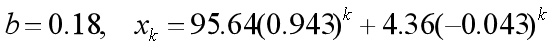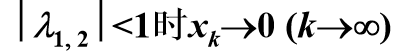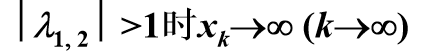植物能够一直繁殖下去的条件为b>0.191

# 线性常系数差分方程组

## 汽车租赁公司的运营

### 问题提出

• 在A市租赁在A, B, C市归还的比例分别为0.6, 0.3, 0.1
• 在B市租赁在A, B, C市归还的比例分别为0.2, 0.7, 0.1
• 在C市租赁在A, B, C市归还的比例分别为0.1, 0.3, 0.6

### 模型建立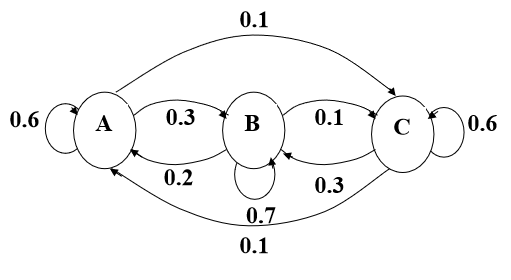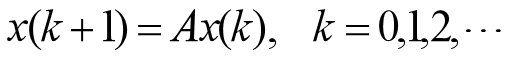观察n年以后的3个城市的汽车数量变化情况

### 模型求解

>> A=[0.6,0.2,0.1;0.3,0.7,0.3;0.1,0.1,0.6];
>> n=10;
>> for k=1:n
x(:,1)=[200,200,200]';
x(:,k+1)=A*x(:,k);
end
>>  x(:,k+1)
ans =

179.9324
299.9895
120.0781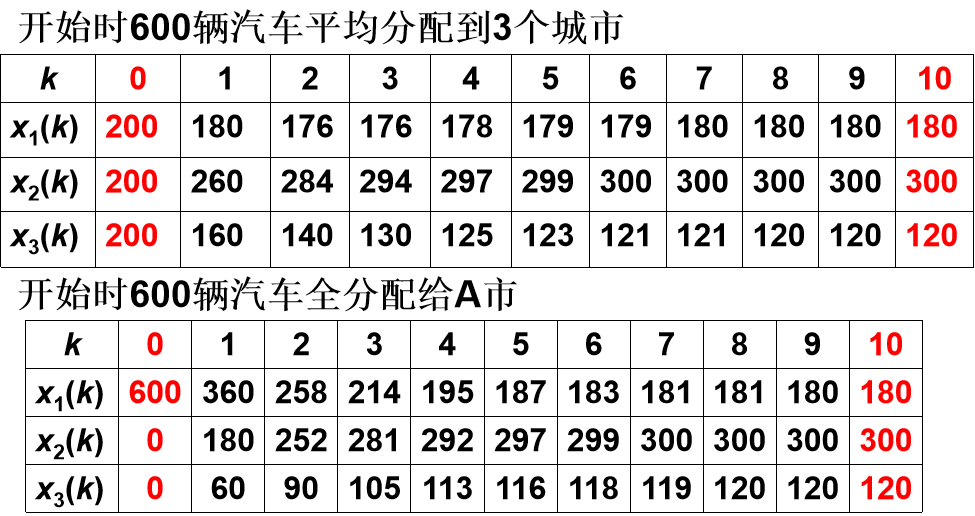时间充分长后3个城市的汽车数量趋向稳定，稳定值与初始分配无关

function x=qczl(n)
A=[0.6,0.2,0.1;0.3,0.7,0.3;0.1,0.1,0.6];
x(:,1)=[200,200,200]';
for k=1:n
x(:,k+1)=A*x(:,k);
end


>> y1=qczl(5);
>> k=0:5;
>> plot(k,y1)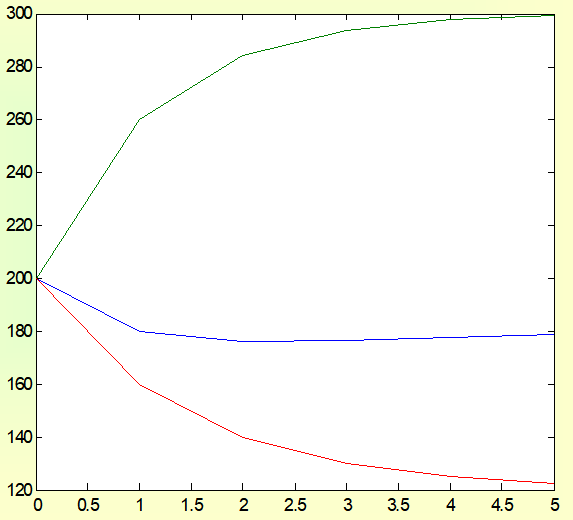## 按年龄分组的种群增长

### 模型建立

• 种群按年龄大小等分为n个年龄组，记i=1,2,…n
• 时间离散为时段，长度与年龄组区间相等，记k=1,2,…
• 第i 年龄组1雌性个体在1时段内的繁殖率为
• 第i 年龄组在1时段内的死亡率为，存活率为

xi(k)~时段k第i 年龄组的种群数量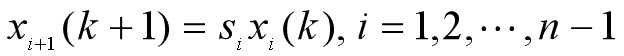### 模型求解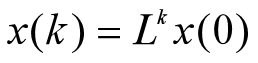>> b=[0,0.2,1.8,0.8,0.2];
>> E=diag([0.5,0.8,0.8,0.1]);
>>  L=[b;E,zeros(4,1)];
>> b=[0,0.2,1.8,0.8,0.2];
>> E=diag([0.5,0.8,0.8,0.1]);
>>  L=[b;E,zeros(4,1)];            %分块矩阵
>> n=30;
>> for k=1:n
x(:,1)=[100,100,100,100,100]';
x(:,k+1)=A*x(:,k);
end

ans =
434.1877
211.4436
164.6266
128.9265
12.5635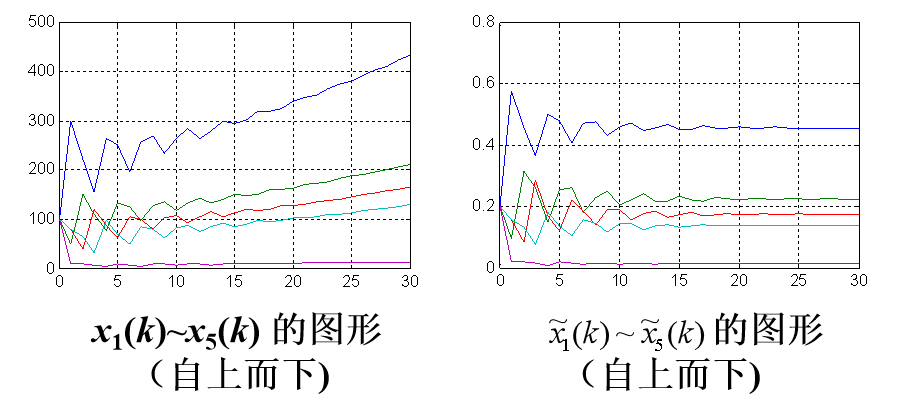# 差分方程求解方法

## 迭代法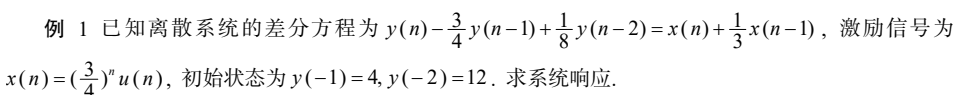根据激励信号和初始状态，手工依次迭代可算出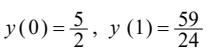利用 MATLAB 中的 filter 函数实现迭代过程的 m 程序如下:

clc;clear;format compact;
a=[1,-3/4,1/8],b=[1,1/3,0], %输入差分方程系数向量,不足补0对齐
n=0:10;xn=(3/4).^n, %输入激励信号
zx=[0,0],zy=[4,12], %输入初始状态
zi=filtic(b,a,zy,zx),%计算等效初始条件
[yn,zf]=filter(b,a,xn,zi),%迭代计算输出和后段等效初始条件

MATLAB提供的 filter函数是一个内建函数， 用 type命令看不到程序代码。为了理解迭代思想，下面根据图 所示的直接Ⅰ型结构， 重写实现迭代法的 m 程序。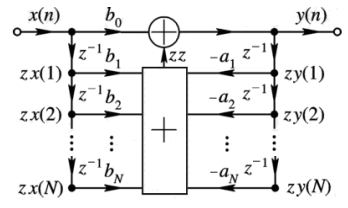clc;clear;format compact;
a=[1, -3/4, 1/8], b=[1, 1/3, 0], %输入差分方程系数向量, 不足补 0 对齐
n=0:10;x=(3/4).^n, %输入激励信号
zx=[0, 0], zy=[4, 12], %输入初始状态
% 下面是按直接Ⅰ型结构迭代的通用程序 %
N=length(a)-1, %计算数据存储长度
L=length(x), %计算激励信号长度
y=zeros(1, L);%输出信号初始化
for i=1:L; %逐个计算输出信号
for n=1:N;z(n)=b(n+1)*zx(n)-a(n+1)*zy(n);end %分算过去
zz=sum(z);%计算输出中的过去分量
y(i)=b(1)*x(i)+zz;% 计算当前输出 y(n)
for n=N:-1:2, zx(n)=zx(n-1);zy(n)=zy(n-1);end%过去数据下移
zx(1)=x(i);zy(1)=y(i);%当前的激励和输出变为过去, 以便算下一个输出
end
%理解 filter 函数中 zf 参数的意义
zf=zeros(1, N);%初始化 zf
for k=1:N; %逐个计算 zf 参数
for n=1:N;z(n)=b(n+1)*zx(n)-a(n+1)*zy(n);end %算 z(n)
zf(k)=sum(z);% 计算第 k 个 zf
for k=N:-1:2, zx(k)=zx(k-1);zy(k)=zy(k-1);end;%过去数据下移
zx(1)=0;zy(1)=0;% 没有当前的激励和输出变为过去, 算下一个 zf
end
y, zf, %显示输出和 zf 参数

## 时域经典法

• (1) 求齐次解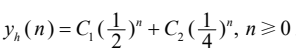•  (2) 求方程的特解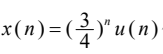代入差分方程右端得自由项为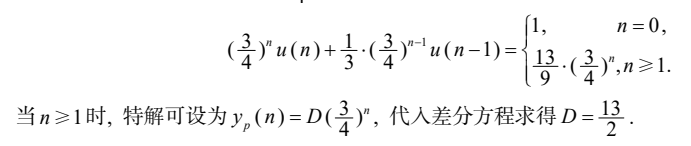•  (3) 利用边界条件求完全解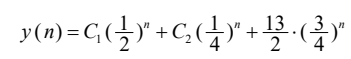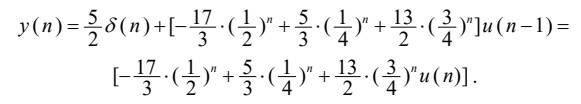MATLAB没有专用的差分方程求解函数，但可调用maple符号运算工具箱中的rsolve函数实现，格式为

y=maple('rsolve({equs, inis},y(n))')

• equs为差分方程表达式
• inis为边界条件
• y(n)为差分方程中的输出函数式

clc;clear;format compact;
yn=maple('rsolve({y(n)-3/4*y(n-1)+1/8*y(n-2)=(3/4)^n+1/3*(3/4)^(n-1),y(0)=5/2,y(-1)=4},y(n))'),
hn=maple('rsolve({y(n)-3/4*y(n-1)+1/8*y(n-2)=0,y(0)=1,y(1)=13/12},y(n))'),

## 双零法

• 零输入响应是激励为零，由系统的初始状态所产生的响应；零输入响应要求差分方程右端为零，故特解为零；完全解为齐次解形式，系数可直接由初始状态确定。
• 零状态响应是初始状态为零，由激励信号所产生的响应。计算零状态响应可用时域经典法，也可用卷积法。

yzi=maple('rsolve({y(n)-3/4*y(n-1)+1/8*y(n-2)=0,y(-1)=4, y(-2)=12},y(n))'),
yzs=maple('rsolve({y(n)-3/4*y(n-1)+1/8*y(n-2)=(3/4)^n+1/3*(3/4)^(n-1),y(0)=1, y(-1)=0},y(n))'),

04-03
05-18
04-294037
08-045301
09-022081
08-052806
02-22849
04-281万+
05-27355
02-08987
07-062万+
08-063843
07-284232
08-195721
07-281927
05-241万+
03-165807
03-16444
05-071万+
09-106110

### “相关推荐”对你有帮助么？

•非常没帮助
•没帮助
•一般
•有帮助
•非常有帮助

©️2022 CSDN 皮肤主题：猿与汪的秘密 设计师：我叫白小胖被折叠的  条评论 为什么被折叠?到【灌水乐园】发言¥2 ¥4 ¥6 ¥10 ¥20余额支付 (余额：-- )扫码支付获取中扫码支付点击重新获取扫码支付1.余额是钱包充值的虚拟货币，按照1:1的比例进行支付金额的抵扣。
2.余额无法直接购买下载，可以购买VIP、C币套餐、付费专栏及课程。余额充值